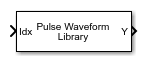# Pulse Waveform Library

Library of pulse waveforms

• Library:

•## Description

The Pulse Waveform Library generates different types of pulse waveforms from a library of waveforms.

## Ports

### Input

expand all

Index to select the waveform, specified as a positive integer. The index selects the waveform from the set of waveforms defined by the Specification of each waveform in the library parameter.

Data Types: `double`

### Output

expand all

Pulse waveform samples, returned as a complex-valued vector or complex-valued matrix.

Data Types: `double`
Complex Number Support: Yes

## Parameters

expand all

Sample rate of the output waveform, specified as a positive scalar. The ratio of Sample rate (Hz) to each element in the Pulse repetition frequency (Hz) vector must be an integer. This restriction is equivalent to requiring that the pulse repetition interval is an integral multiple of the sample interval.

Pulse waveforms, specified as a cell array. Each cell of the array contains the specification of one waveform. Each waveform is also a cell array containing the parameters of the waveform.

`{{Waveform 1 Specification},{Waveform 2 Specification},{Waveform 3 Specification}, ...}`
This block supports four built-in waveforms and also lets you specify custom waveforms. Each built-in waveform specifier consists of a waveform identifier followed by several name-value pairs that set the properties of the waveform.

Built-in Waveforms

 Waveform type Waveform identifier Waveform name-value pair arguments Linear FM `'LinearFM'` See Linear FM Waveform Arguments Phase coded `'PhaseCoded'` See Phase-Coded Waveform Arguments Rectangular `'Rectangular'` See Rectangular Waveform Arguments Stepped FM `'SteppedFM'` See Stepped FM Waveform Arguments

You can create a custom waveform with a user-defined function. The first input argument of the function must be the sample rate. Use a function handle instead of the waveform identifier in the first cell of a waveform specification. The remaining cells contain all function input arguments except the sample rate. Specify all input arguments in the order they are passed into the function. The function must have at least one output argument to return the samples of each pulse in a column vector. You can only create custom waveforms when you set Simulate using to ```Interpreted Execution```.

Source of simulation sample time, specified as ```Derive from waveform parameters``` or `Inherit from Simulink engine`. When set to `Derive from waveform parameters`, the block runs at a variable rate determined by the PRF of the selected waveform. The elapsed time is variable. When set to `Inherit from Simulink engine`, the block runs at a fixed rate so the elapsed time is a constant.

#### Dependencies

To enable this parameter, select the Enable PRF selection input parameter.

Block simulation, specified as `Interpreted Execution` or ```Code Generation```. If you want your block to use the MATLAB® interpreter, choose `Interpreted Execution`. If you want your block to run as compiled code, choose `Code Generation`. Compiled code requires time to compile but usually runs faster.

Interpreted execution is useful when you are developing and tuning a model. The block runs the underlying System object™ in MATLAB. You can change and execute your model quickly. When you are satisfied with your results, you can then run the block using ```Code Generation```. Long simulations run faster with generated code than in interpreted execution. You can run repeated executions without recompiling, but if you change any block parameters, then the block automatically recompiles before execution.

This table shows how the Simulate using parameter affects the overall simulation behavior.

When the Simulink® model is in `Accelerator` mode, the block mode specified using Simulate using overrides the simulation mode.

Acceleration Modes

 Block Simulation Simulation Behavior `Normal` `Accelerator` `Rapid Accelerator` `Interpreted Execution` The block executes using the MATLAB interpreter. The block executes using the MATLAB interpreter. Creates a standalone executable from the model. `Code Generation` The block is compiled. All blocks in the model are compiled.

## Extended Capabilities

### C/C++ Code GenerationGenerate C and C++ code using Simulink® Coder™.